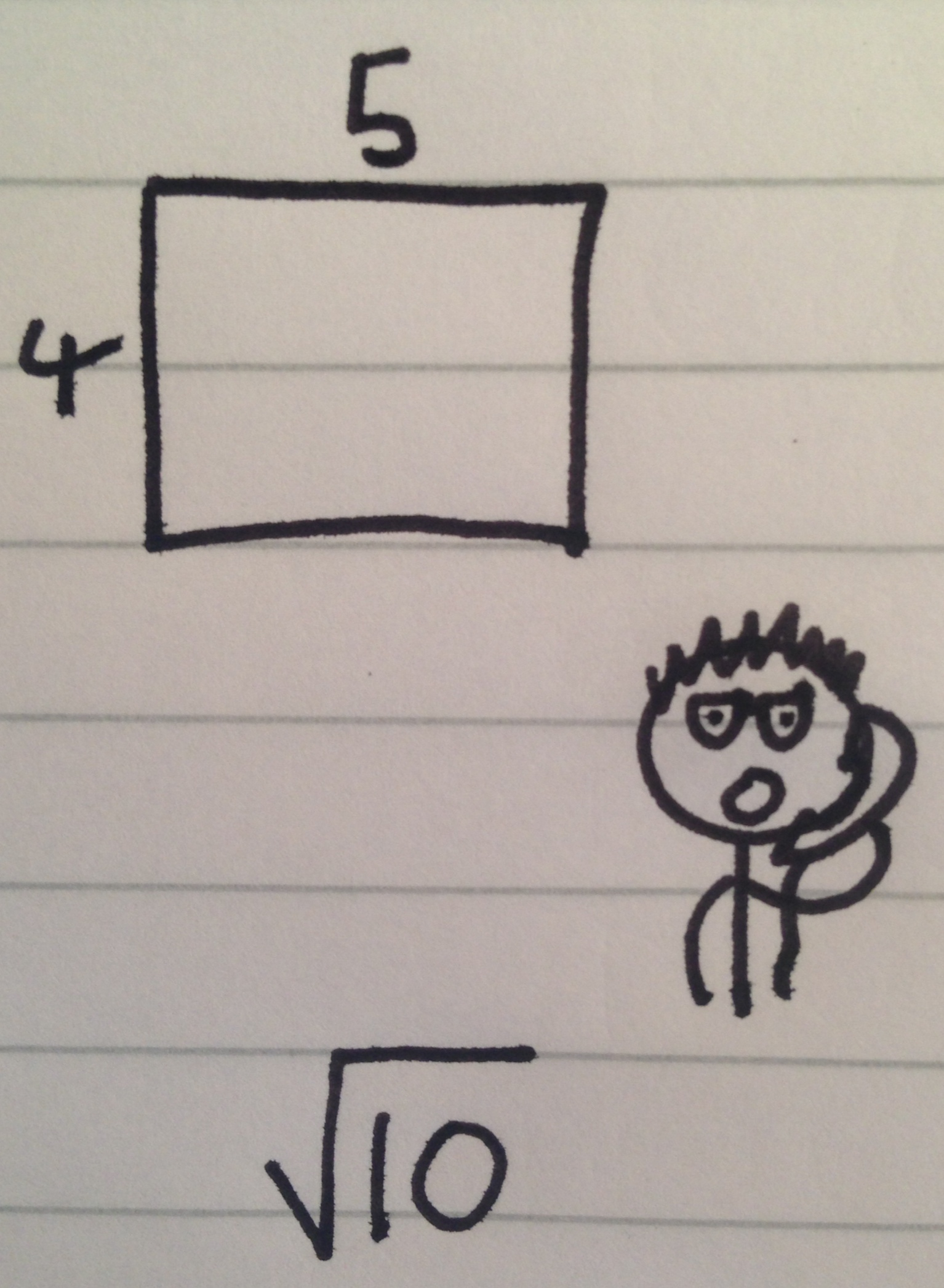# The Wrecked Rectangle

Geometry Level 4A mathematician has a rectangle of length 5, width 4. His physicist friend phones him up with a problem involving this rectangle, but the sides have been scaled down by a factor of $\sqrt { 10 }$. The physicist asks the mathematician to determine the area of the rectangle, for her to use in her calculations.

The mathematician does the calculation, but decides to test the physicist and gives his answer in the form:

$\sqrt { x\sqrt { y\sqrt { x\sqrt { y\sqrt { x\sqrt { y\sqrt { x... } } } } } } }.$

where $x$ and $y$ are positive integers.

What is the sum of all possible values of x and y that the mathematician could have given to the physicist?

×

Problem Loading...

Note Loading...

Set Loading...